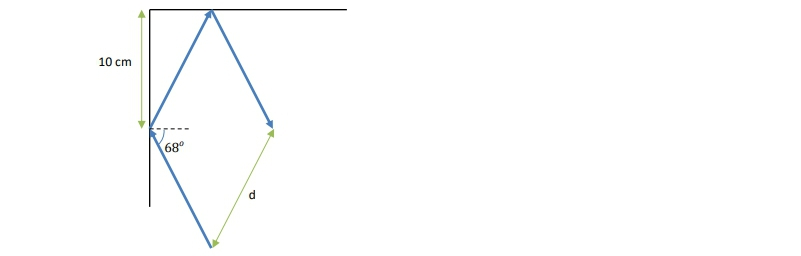## Physics

Learn the toughest concepts covered in Physics with step-by-step video tutorials and practice problems by world-class tutors

33. Geometric Optics

# Reflection Of Light

1
concept

## Reflection of Light5m
Play a video:
Hey, guys, in this video, we're going to talk about the reflection of light off of a boundary between two media. Okay, let's get to it. Now. Remember when a wave encounters a boundary, it could do one of two things. It can either reflect off of that boundary or it can transmit through that boundary and propagate in the new media. In reality waves, they're gonna do a little bit of both now for light boundaries. Air, typically referred to or media, I should say, are typically referred to as one of two things. They could either be reflective, like the surface of the mirror, and mainly on Lee. Allow reflection. Or they could be transparent like glass and mainly allow transmission. Okay, for now, we wanna we wanna talk about reflective surfaces. Alright, Light reflects off of a boundary in the same manner as a ball undergoing an elastic collision with a wall. Okay, if we have a ball at some point with some momentum right here in some direction, when it hits the wall, it's going to conserve that momentum, but it's going to go in the opposite direction, okay? And it's gonna enter with some angle and it's gonna leave with some other angle light is gonna do the same thing if I draw a ray of light encountering a boundary between two media that Ray is gonna leave at some angle. Okay, We have something called the Law of Reflection, which holds for the elastic collision. Just like it holds for reflection of light. That states that that reflected angle is actually the same as the incident angle, the angle with which it hits the boundary. Okay, now, just a side note. This isn't gonna be important right now, but this is gonna be very important later. Whenever light encounters a boundary, we always measure the angle relative to the normal direction relative to some line that is perpendicular to the boundary. Right. This is the normal. Just like the normal force is perpendicular. The normal direction is perpendicular to a surface. Okay, let's do an example. We want to find the missing angle theta using the law of reflection. Okay, so this light ray is incident at 65 degrees. It undergoes reflection right here, which means that the outgoing angle is also going to be 65 degrees. That's just what the law reflection says now notice this is the normal too sorry, Normal to this surface right? Which means that it's had a 90 degree angle from the surface. So this angle right here is gonna be the complementary angle to degrees, which is 25 degrees, right, because they have to add up to 90. Now notice we have a triangle right here that looks like this. Here's our 25 degree angle. Here's our 125 degree angle and here is an unknown angle. Whoops, letting men wise myself remember that the sum of all of the internal angles within a triangle has to be so 25 degrees plus 120 degrees plus this unknown angle has to be 180 degrees. That means that this unknown angle is actually 35 degrees. Okay, so that tells us what this angle is. This angle is 35 degrees. Now, once again, this line right here is the normal to the second surface, which means that it's perpendicular to the second surface. So this angle right here is going to be the complementary angle to 35 degrees right, the angle that, when added to 35 degrees, equals 90 and that is 55 degrees. So what is theta have to be, while the law of reflection says it has to be the same as that incident angle which on the second surface is 55 degrees, So theta, therefore, is 55 degrees. Alright, guys, that wraps up our discussion on the reflection of light and the law reflection. Thanks for watching.
2
example

## Hanging Mirror4m
Play a video: